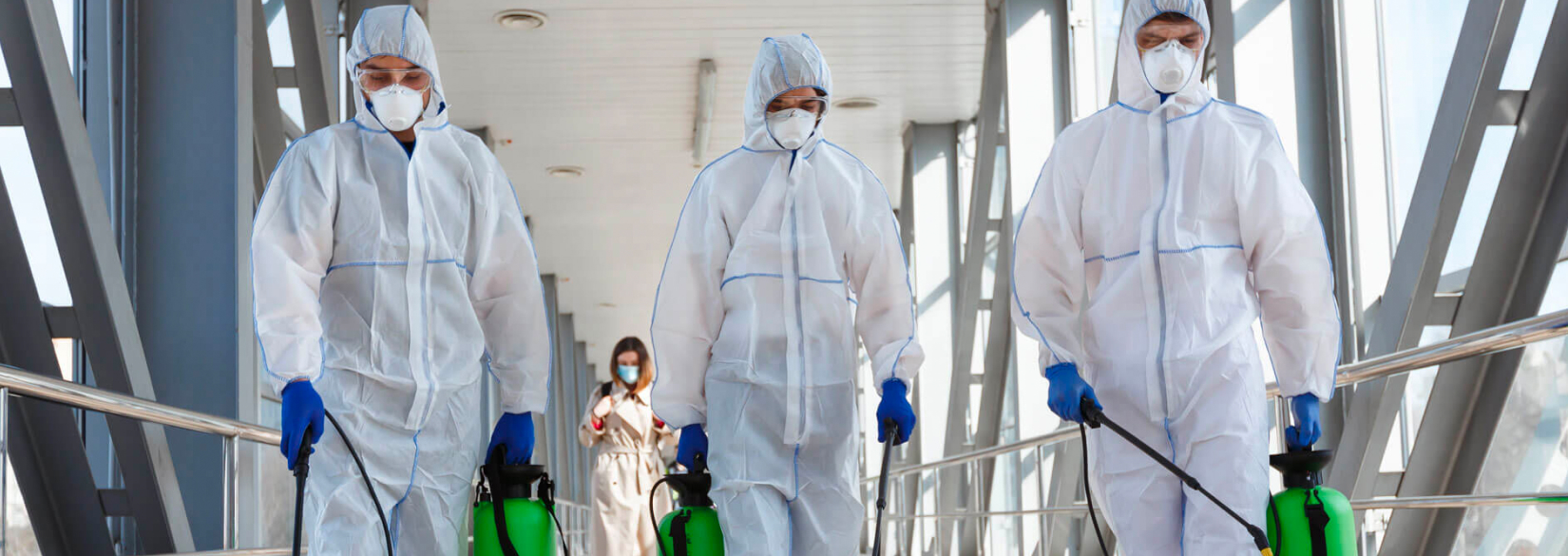# Case Study

## Triple O-Zone TechnologyOur environment is filled with a variety of pathogens that can potentially compromise our immune system and put your health and the health of your employees at risk.## Problem

Here is where you add the problems. Here is where you add the problems. Here is where you add the problems. Here is where you add the problems. Here is where you add the problems. Here is where you add the problems. Here is where you add the problems.## Solution

Here is where you add the problems. Here is where you add the problems. Here is where you add the problems. Here is where you add the problems. Here is where you add the problems. Here is where you add the problems. Here is where you add the problems.## Outcome

Here is where you add the problems. Here is where you add the problems. Here is where you add the problems. Here is where you add the problems. Here is where you add the problems. Here is where you add the problems. Here is where you add the problems.## Website Design and Development

You can add the contents for this project here.

### Logo

You can add the contents for this project here. You can add the contents for this project here. You can add the contents for this project here. You can add the contents for this project here. You can add the contents for this project here.